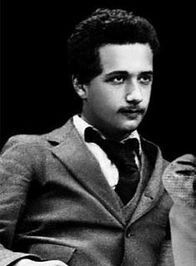## Tuesday, 25 February 2020

### Einstein's equationEinstein age 18. Credit.
We are now on section 4.2 which has some very shady approximations. However we do get to Einstein's equation for general relativity if we tolerate that. The equation is$$R_{\mu\nu}-\frac{1}{2}Rg_{\mu\nu}=8\pi GT_{\mu\nu}$$where $R_{\mu\nu},R$ are the Ricci tensor and scalar which tell us about the curvature of spacetime, $g_{\mu\nu}$ is the metric, $G$ is Newton's constant and $T_{\mu\nu}$ is the energy-momentum tensor. So the equation tells us how the curvature of spacetime reacts to the presence of energy-momentum (which includes mass). Newton is not forgotten altogether😊.

The equation can also be written as $$R_{\mu\nu}=8\pi G\left(T_{\mu\nu}-\frac{1}{2}Tg_{\mu\nu}\right)$$where $T=g_{\mu\nu}T_{\mu\nu}$ and in empty space where $T_{\mu\nu}=0$ that gives us$$R_{\mu\nu}=0$$The equation is a field equation for the metric and the Newtonian gravity field equation is Poisson's equation$$\nabla^2\Phi=4\pi G\rho$$where $\Phi$ is the gravitational potential and $\rho$ the mass density.

The section starts by plausibly guessing that GR field equation must be of the form $$R_{\mu\nu}-\frac{1}{2}Rg_{\mu\nu}=\kappa T_{\mu\nu}$$where $\kappa$ is a constant we must find. The GR field equation must be the same as Poisson's equation in almost-flat spacetime. So we use a small perturbation $h_{\mu\nu}$ on the flat metric: $$g_{\mu\nu}=\eta_{\mu\nu}+h_{\mu\nu}$$and discarding second order terms in $h_{\mu\nu}$ eventually work out that $\kappa=8\pi G$ to bring the two equations into line.

However! Carroll's 4.38 is wrong. It says $T_{00}=\rho$ and in fact $T_{00}=\rho\left(1-h_{00}\right)$ and  if 4.38 were right then 4.39 would be wrong, but in fact it is right. Carroll is sort of having it both ways and we only get $\ \kappa\approx8\pi G$ at best. It contains first order terms in $h_00$. Hopefully the next section using the Lagrangian formulation will do better!

See Commentary 4.2 Einsteins equation.pdf (5 pages) for the details.